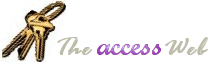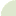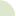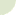Home  |   French  |   About  |   Search  | mvps.orgWhat's New Table Of Contents Credits Netiquette 10 Commandments  Bugs Tables Queries Forms Reports Modules APIs Strings Date/Time General Downloads Resources Search Feedback mvps.orgStrings: Parsing character separated string into individual components Author(s) Dev Ashish(Q) I have string which contains values separated by a comma/colon/semi colon/space. How can I extract each value from that string? (A) You can use these two functions provided by Microsoft to retrieve each value. Note that the functions are written for comma separated values but can easily be modified to work with any other character. Use the Sub Test as an example ```'******************* Code Start **************** Function CountCSWords(ByVal s) As Integer 'Counts the words in a string that are separated by commas. Dim WC As Integer, Pos As Integer If VarType(s) <> 8 Or Len(s) = 0 Then CountCSWords = 0 Exit Function End If WC = 1 Pos = InStr(s, ",") Do While Pos > 0 WC = WC + 1 Pos = InStr(Pos + 1, s, ",") Loop CountCSWords = WC End Function Function GetCSWord(ByVal s, Indx As Integer) 'Returns the nth word in a specific field. Dim WC As Integer, Count As Integer Dim SPos As Integer, EPos As Integer WC = CountCSWords(s) If Indx < 1 Or Indx > WC Then GetCSWord = Null Exit Function End If Count = 1 SPos = 1 For Count = 2 To Indx SPos = InStr(SPos, s, ",") + 1 Next Count EPos = InStr(SPos, s, ",") - 1 If EPos <= 0 Then EPos = Len(s) GetCSWord = Trim(Mid(s, SPos, EPos - SPos + 1)) End Function Sub Test() Dim strAString As String Dim I As Integer Dim intCnt As Integer strAString = "This,calls,the,two,functions,listed,above" 'Find out how many comma separated words 'are present intCnt = CountCSWords(strAString) 'Now call the other function to retrieve each one in turn For I = 1 To intCnt Debug.Print GetCSWord(strAString, I) Next End Sub '******************* Code End ****************  ```© 1998-2010, Dev Ashish & Arvin Meyer, All rights reserved. Optimized for Microsoft Internet Explorer